# Regression Analysis: Variable’s affair!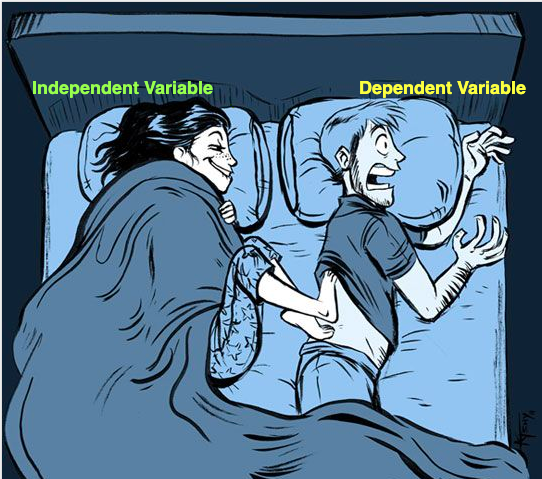Cause and Effect relationship

# Discovering the affair of Independent and dependent variables.

## Regression Analysis Types

One size doesn’t fit all!

Depending on conditions and data characteristics, a particular regression model is suited more than others. Regression algorithms tries to model the “relationship” between variables. To make precise prediction (or accurate modeling of the data), algorithms minimize the error in predictions. So it won’t be incorrect to see regression more as process.

Some of the commonly used regression models are:

• Linear Regression
Linear regression is the most known and possibly oldest algorithm in statistics and machine learning. The model is useful for the data where the input variables and output variable share linear relationship. When the relationship between single input variable and output variable is analysed, it is known as simple linear regression. One unit change in input variable causes change in output variable by a unit. In case of multiple input variables, it is known as multiple linear regression.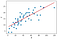Simple Linear regression model. source
• Logistic Regression
Logistic regression is named for using logistic function (sigmoid) as underlying method. Logistic regression is widely used in binary classification problems. However, in case of regression problem it works on probability of occurence or non-occurence of an event. Logistic regression is different from the linear regression as it do not assume/require linear relationship between dependent and independent variable.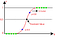Logistic regression model. source
• Polynomial Regression
Polynomial literal means multiple terms (constants, variables, and exponents). A polynomial equation can have combinations of constants, variables and exponents. When the best fit line is non-linear or in other words the data is not linearly separable, Polynominal regression comes to the rescue.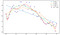Polynomial regression. source
• Ridge Regression
Ridge regression follows the idea of ordinary least square method but also adds a small bias to reduce the variance. Ridge regression adjust the slope of fit line. Ridge regression attempts to reduce the model complexity and therefore tackles overfitting.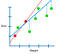Ridge regression model. source
• Stepwise Regression
This is very powerful method when there are multiple independent variables and we want to screen the most important variables. As the name suggest this is step by step process where the variables are added or removed based on p-value (t-statistics). This is a more statistical approach to select most relevant independent variables.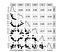Stepwise regression. source
• Lasso Regression (Least Absolute Shrinkage and Selection Operator)
Lasso follows the same idea as the ridge regression to add cost function. The difference is lasso uses absolute value of bias instead of squared bias. Lasso can be used to handle the overfitting as well as independent variable selection.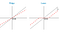Ridge and Lasso regression best fit line. source
• Multivariate Adaptive Regression Splines (MARS)
MARS is nonparametric statistical method and it does not make assumptions about the independent and dependent variable relationship. The idea is to divide the data into splines(segments) of different slopes. The splines slopes (basis functions- BFs) are connected and results into the flexible model capable of handling linear and non-linear relationship.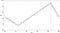Simple Mars algorithm. source
• Locally Estimated Scatterplot Smoothing (LOESS)
LOESS also known as Locally weighted scatterplot smoothing (LOWESS) is a technique used to fit a smooth line to a scatter plot when the data is sparse, noisy, or have feeble inter-relationships.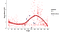LOESS regression model. source

# Anecdote time!Ursa Major constellation

Physics changed the way I appreciate the stars, but data science changed the way I perceive the stars.

From sketching the patterns by connecting dots (stars) and searching for constellations, recently I caught myself apprehending stars as data points. I was observing Ursa major and said to myself this is typical model over fitting scenario! What would be the best fit line?

How did data science changed your perspective?
I would love to learn your anecdote of data science.

If you find this post helpful please let me know by comment section. You can also send me your suggestions and topics of your interest.

References:

I am a data science enthusiast and aiming to continue enhancing the art of discovering and telling data-stories hidden in plain sight.

## More from Nisha Chauhan

I am a data science enthusiast and aiming to continue enhancing the art of discovering and telling data-stories hidden in plain sight.